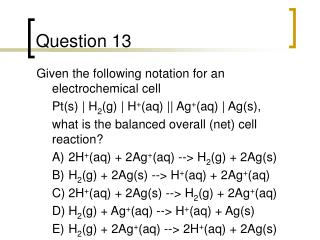DownloadDownload PresentationQuestion 13

# Question 13

Télécharger la présentation## Question 13

- - - - - - - - - - - - - - - - - - - - - - - - - - - E N D - - - - - - - - - - - - - - - - - - - - - - - - - - -
##### Presentation Transcript

1. Question 13 • Given the following notation for an electrochemical cell • Pt(s) | H2(g) | H+(aq) || Ag+(aq) | Ag(s), • what is the balanced overall (net) cell reaction? • A) 2H+(aq) + 2Ag+(aq) --> H2(g) + 2Ag(s) • B) H2(g) + 2Ag(s) --> H+(aq) + 2Ag+(aq) • C) 2H+(aq) + 2Ag(s) --> H2(g) + 2Ag+(aq) • D) H2(g) + Ag+(aq) --> H+(aq) + Ag(s) • E) H2(g) + 2Ag+(aq) --> 2H+(aq) + 2Ag(s)

2. Question 14 • Consider an electrochemical cell constructed from the following half cells, linked by an • external circuit and by a KCl salt bridge. • - an Al(s) electrode in 1.0 M Al(NO3)3 solution • - a Pb(s) electrode in 1.0 M Pb(NO3)2 solution • The balanced overall (net) cell reaction is • A) Pb(s) + Al3+(aq) --> Pb2+(aq) + Al(s.) • B) 3Pb(s) + 2Al3+(aq) --> 3Pb2+(aq) + 2Al(s). • C) 3Pb2+(aq) + 2Al(s) --> 3Pb(s) + 2Al3+(aq). • D) Pb2+(aq) + Al(s) --> Pb(s) + Al3+(aq).

3. Question 15 • Consider an electrochemical cell constructed from the following half cells, linked by a KCl • salt bridge. • - an Al(s) electrode in 0.5 M Al2(SO4)3 solution • - a Pb(s) electrode in 1.0 M Pb(NO3)2 solution • Which electrode is the anode? • A) Al • B) Pb • C) Neither

4. Question 16 • Consider an electrochemical cell constructed from the following half cells, linked by a KCl salt bridge. • - a Fe electrode in 1.0 M FeCl2 solution • - a Sn electrode in 1.0 M Sn(NO3 )2 solution • When the cell is running spontaneously, which choice includes only true statements and no false ones? • A) The tin electrode loses mass and the tin electrode is the cathode. • B) The tin electrode gains mass and the tin electrode is the cathode. • C) The iron electrode gains mass and the iron electrode is the anode. • D) The iron electrode loses mass and the iron electrode is the cathode. • E) The iron electrode gains mass and the iron electrode is the cathode.

5. Question 18 • A certain electrochemical cell has for its cell reaction: • Zn + HgO --> ZnO + Hg • Which is the half-reaction occurring at the anode? • A) HgO + 2e- --> Hg + O2- • B) Zn2+ + 2e- --> Zn • C) Zn --> Zn2+ + 2e- • D) ZnO + 2e- --> Zn

6. Question 19 • Calculate the value of Eocell for the following reaction: • 2Au(s) + 3Ca2+(aq) --> 2Au3+(aq) + 3Ca(s) • A) -4.37 V • B) -1.37 V • C) -11.6 V • D) 1.37 V • E) 4.37 V

7. Question 20 • Calculate Eocell for a silver-aluminum cell in which the cell reaction is • Al(s) + 3Ag+(aq) --> Al3+(aq) + 3Ag(s) • A) -2.46 V • B) 0.86 V • C) -0.86 V • D) 2.46 V • E) none of these

8. C A A D A B D E D E D C E C A B B C A D Answer Key – Chapter 19